# LineFlow: Simple NLP Dataset Handler for PyTorch or Any FrameworkLineFlow: Simple NLP Dataset Handler for PyTorch or Any Framework

For an NLP task, you might need to tokenize text or build the vocabulary in the pre-processing. And you probably have experienced that the pre-processing code is as messy as your desk. Forgive me if your desk is clean :) I have such experience too. That’s why I create LineFlow to ease your pain! It will make your “desk” as clean as possible. How does the real code look like? Take a look at the figure below. The pre-processing including tokenization, building the vocabulary, and indexing.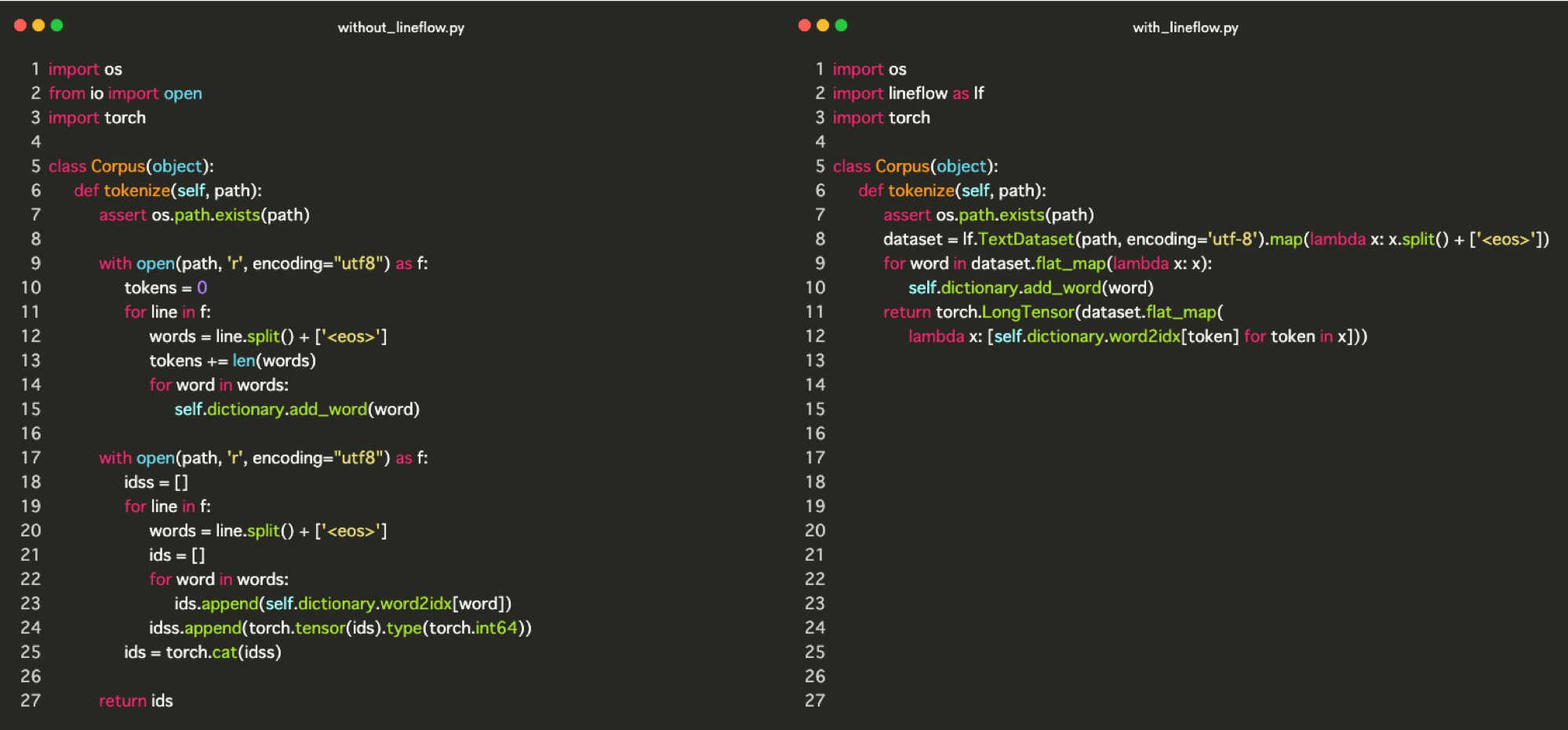I used Codeimg.io for this picture.

The left part is the example code from the PyTorch official examples repository, which does common pre-processing on text data. The right part is written with LineFlow to implement the exact same processing. You should get the idea that how LineFlow easies your pain. You can check the full code from this link.

In this post, I will explain the right-part code in detail and show you the usage of LineFlow. Let’s get started for a clean “desk” life!

Loading the text data is done by line 8 on the code above. I’ll explain the map later. lf.TextDataset takes the path to the text file as the argument and loads it.

```dataset = lf.TextDataset(path, encoding='utf-8').map(...)
```

The data format that lf.TextDataset expects is each line corresponds to the one data. If your text data satisfies this condition, you can load any kind of text data.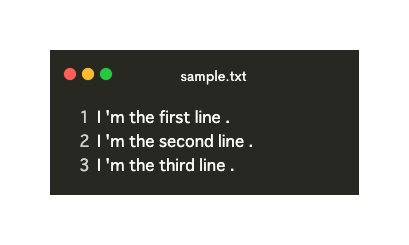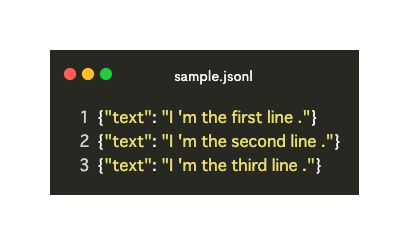After loading, it converts the text data to the list. The item in the list corresponds to the line in the text data. Look at the following figure. This is the intuitive image for lf.TextDataset. The d in the figure stands for dataset in the code.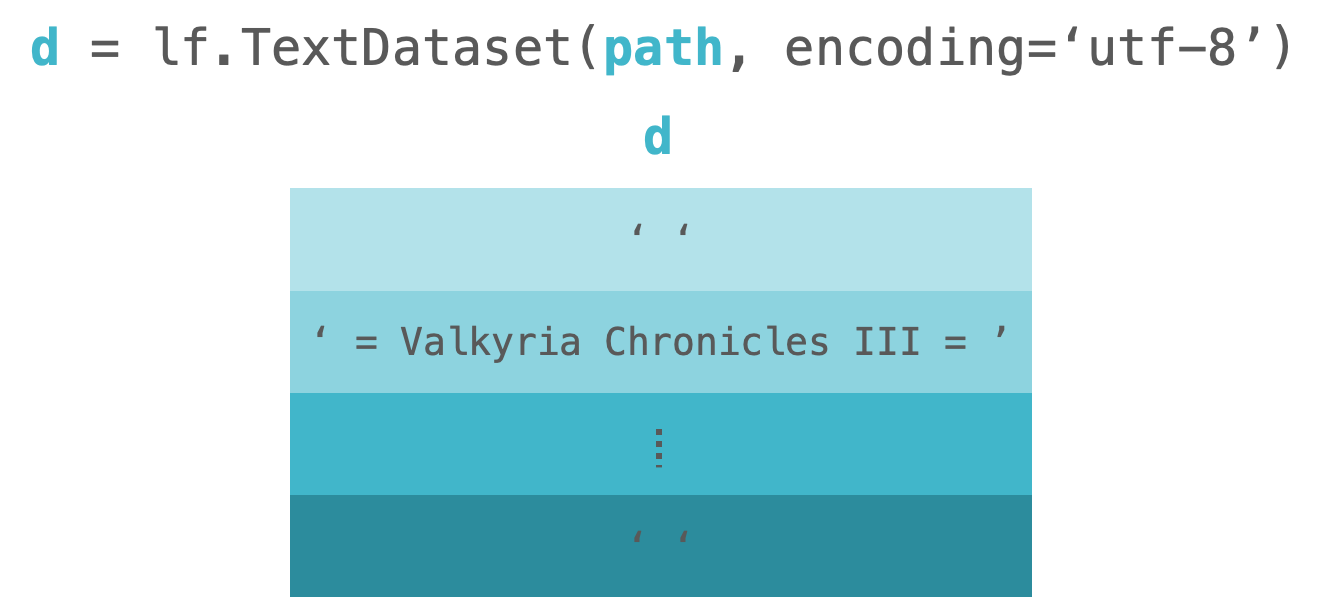LineFlow has already provided some of the publicly available datasets. So you can use it immediately. You can check the provided datasets here.

# 2. Tokenization

Text tokenization is also done by line 8. `map` apply the processing passed as the argument to each line of the text data.

```dataset = lf.TextDataset(...).map(lambda x: x.split() + ['<eos>'])
```

Look at the following figure. This is the intuitive image for `lf.TextDataset.map`. The `d` in the figure stands for `dataset` in the code.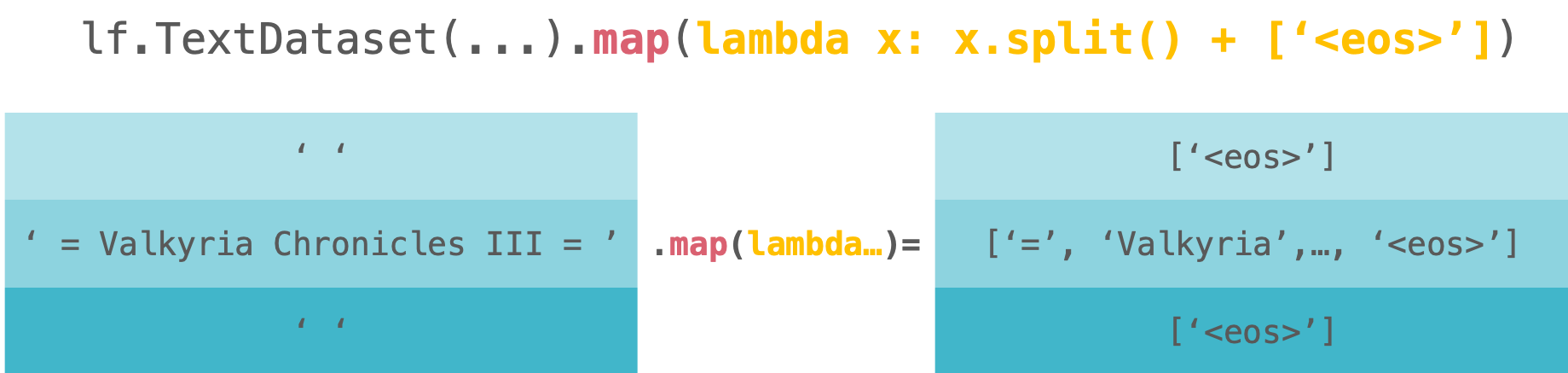Let’s dive into the actual processing below.

```lambda x: x.split() + ['<eos>']
```

Here, we split each line in the text data by the white space to tokens and then add `<eos>` to the end of these tokens. We follow the way of the processing in the WikiText official page.

In this time, we use `str.split` for tokenization. We can use other tokenization methods like spaCyStanfordNLP, and Bling Fire, etc. For example, we’ll get the following code if you want to use Bling Fire.

```>>> from blingfire import text_to_words
>>> d = lf.TextDataset('/path/to/your/text')
>>> d.map(text_to_words).map(str.split)
```

Also, we can do any processing we want as long as our processing takes each line of text data as the argument. For example, we can compute the number of tokens. In the following code, the number of tokens is defined in the second element.

```>>> d = lf.TextDataset('/path/to/text')
>>> d.map(tokenize).map(lambda x: (x, len(x)))
```

This processing is useful when we’d like to make the mask for attention mechanism or LSTM.

# 3. Indexing

Indexing is done by from line 9 to line 12. These lines are shown in the figure below. In this code block, we build the vocabulary and indexing. Let’s look at these in order.

```for word in dataset.flat_map(lambda x: x):
```

First, we’ll see the block of building the vocabulary. In the following code block, we build the vocabulary. `flat_map` applies the processing passed as the argument to each line in the data and then flatten it. So we’ll get the individual token after `dataset.flat_map(lambda x: x)`.

```for word in dataset.flat_map(lambda x: x):
```

Look at the following figure. This is the intuitive image for `dataset.flat_map(lambda x: x)`. The `d` in the figure stands for `dataset` in the code.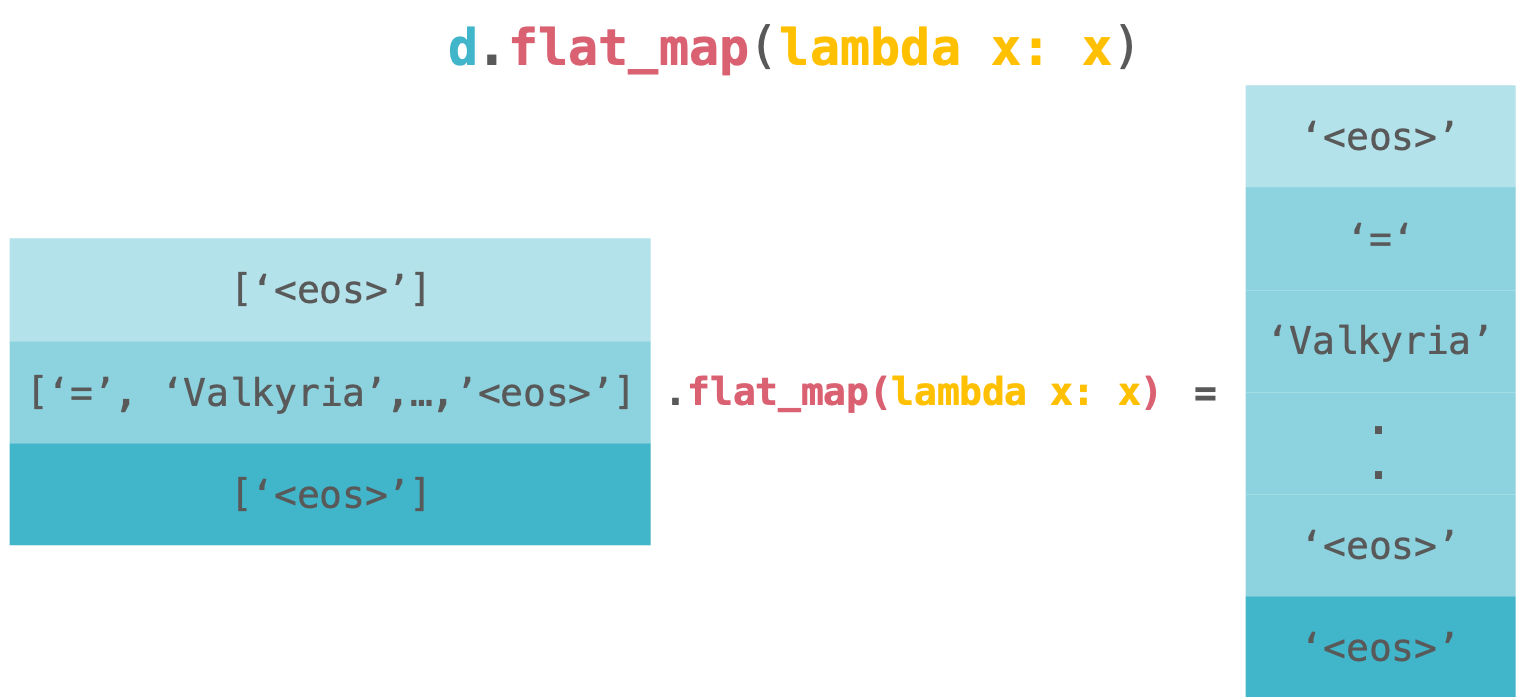`flat_map` is a little bit confusing but it equals to the following code.

```>>> from itertools import chain
>>> chain.from_iterable(map(lambda x: x, dataset))
>>>
>>> dataset.flat_map(lambda x: x) # same as above
```

After extracting each token by using `flatmap, we pass the token to self.dictionary.addword` which build the vocabulary. I don’t explain how it works because it doesn’t relate to this post. But if you are interested in the inside implementation of it, please check this link.

```self.dictionary.add_word(word)
```

Next, we’ll see the code block of indexing. Indexing is done by the following block. Here, we also use`flat_map` to indexing each token and flattening it. This is because PyTorch’s example requires the tensor of the flattened tokens. So we followed it.

```dataset.flat_map(
[lambda x: self.dictionary.word2idx[token] for token in x)])
```

Look at the following figure. This is the intuitive image for `dataset.flat_map(indexer)`. The `d` in the figure stands for `dataset` in the code.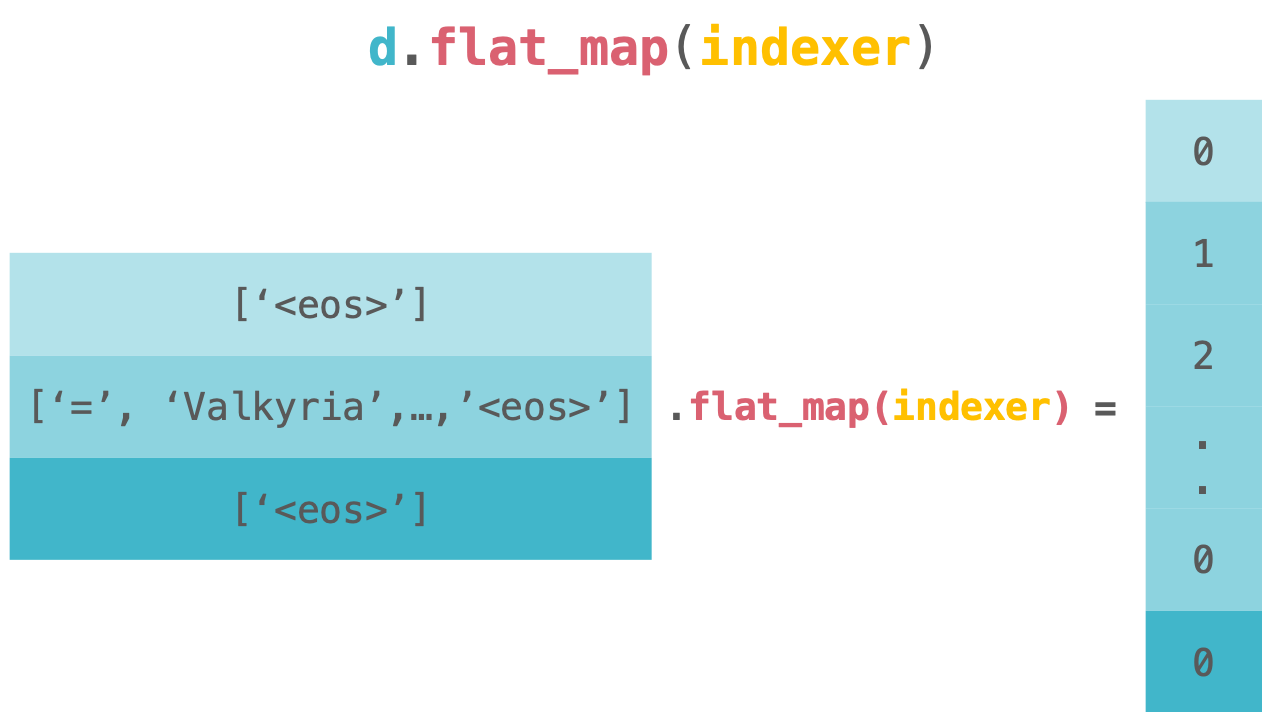This code equals to the following code.

```>>> from itertools import chain
>>> chain.from_iterable(map(indexer, dataset))
>>>
>>> dataset.flat_map(indexer) # same as above
```

Finally, we wrap it by `torch.LongTensor` to make it tensor. We finish loading the text data.

```return torch.LongTensor(dataset.flat_map(...))
```

We can check the full code we’ve seen so far below.

```import os
import torch
import lineflow as lf

class Dictionary(object):
def init(self):
self.word2idx = {}
self.idx2word = []
```def add_word(self, word):
if word not in self.word2idx:
self.idx2word.append(word)
self.word2idx[word] = len(self.idx2word) - 1
return self.word2idx[word]

def __len__(self):
return len(self.idx2word)```
class Corpus(object):
def init(self, path):
self.dictionary = Dictionary()
self.train = self.tokenize(os.path.join(path, 'train.txt'))
self.valid = self.tokenize(os.path.join(path, 'valid.txt'))
self.test = self.tokenize(os.path.join(path, 'test.txt'))
```def tokenize(self, path):
assert os.path.exists(path)
dataset = lf.TextDataset(path, encoding='utf-8').map(lambda x: x.split() + ['&lt;eos&gt;'])
for word in dataset.flat_map(lambda x: x):
lambda x: [self.dictionary.word2idx[token] for token in x]))```
```

That’s all for the explanation. LineFlow completes the less loop and the less nested code by vectorizing the text data. We can do the exact same by using Python’s map. But LineFlow provides us the readable and clean code because it builds the processing like the pipeline (Fluent Interface).

If you like LineFlow and want to know more, please visit the repository below.

https://github.com

============================================

## 50 Data Science Jobs That Opened Just Last Week

Data Science and Analytics market evolves to adapt to the constantly changing economic and business environments. Our latest survey report suggests that as the overall Data Science and Analytics market evolves to adapt to the constantly changing economic and business environments, data scientists and AI practitioners should be aware of the skills and tools that the broader community is working on. A good grip in these skills will further help data science enthusiasts to get the best jobs that various industries in their data science functions are offering.

## Basic Data Types in Python | Python Web Development For Beginners

In the programming world, Data types play an important role. Each Variable is stored in different data types and responsible for various functions. Python had two different objects, and They are mutable and immutable objects.

## Data Science Course in Dallas

Become a data analysis expert using the R programming language in this [data science](https://360digitmg.com/usa/data-science-using-python-and-r-programming-in-dallas "data science") certification training in Dallas, TX. You will master data...

## Applications Of Data Science On 3D Imagery Data

The agenda of the talk included an introduction to 3D data, its applications and case studies, 3D data alignment and more.

## Python For Data Science - How to use Data Science with Python

This Edureka video on 'Python For Data Science - How to use Data Science with Python - Data Science using Python ' will help you understand how we can use python for data science along with various use cases. What is Data Science? Why Python? Python Libraries For Data Science. Roadmap To Data Science With Python. Data Science Jobs and Salary Trends